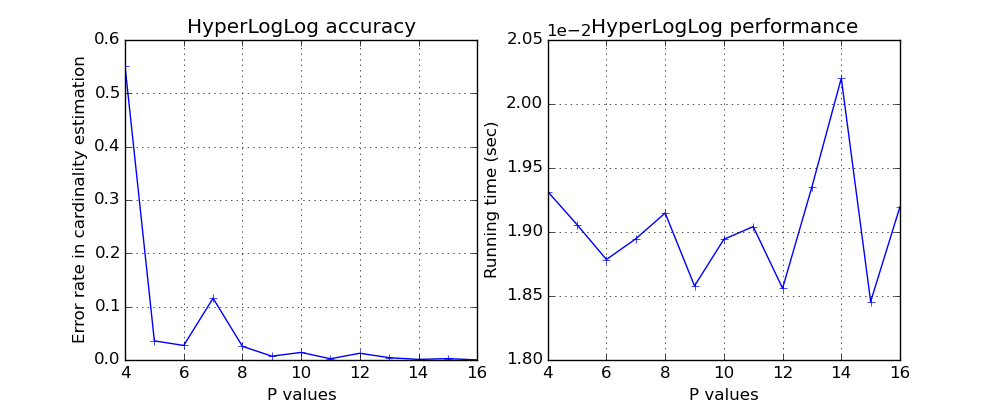# HyperLogLog¶

HyperLogLog is capable of estimating the cardinality (the number of distinct values) of dataset in a single pass, using a small and fixed memory space. HyperLogLog is first introduced in this paper by Philippe Flajolet, Éric Fusy, Olivier Gandouet and Frédéric Meunier.

from datasketch import HyperLogLog

data1 = ['hyperloglog', 'is', 'a', 'probabilistic', 'data', 'structure', 'for',
'estimating', 'the', 'cardinality', 'of', 'dataset', 'dataset', 'a']

h = HyperLogLog()
for d in data1:
h.update(d.encode('utf8'))
print("Estimated cardinality is", h.count())

s1 = set(data1)
print("Actual cardinality is", len(s1))


As in MinHash, you can also control the accuracy of HyperLogLog by changing the parameter p.

# This will give better accuracy than the default setting (8).
h = HyperLogLog(p=12)


Interestingly, there is no speed penalty for using higher p value. However the memory usage is exponential to the p value.As in MinHash, you can also merge two HyperLogLogs to create a union HyperLogLog.

h1 = HyperLogLog()
h2 = HyperLogLog()
h1.update('test'.encode('utf8'))
# The makes h1 the union of h2 and the original h1.
h1.merge(h2)
# This will return the cardinality of the union
h1.count()


# HyperLogLog++¶

HyperLogLog++ is an enhanced version of HyperLogLog by Google with the following changes: * Use 64-bit hash values instead of the 32-bit used by HyperLogLog * A more stable bias correction scheme based on experiments on many datasets * Sparse representation (not implemented here)

HyperLogLog++ object shares the same interface as HyperLogLog. So you can use all the HyperLogLog functions in HyperLogLog++.

from datasketch import HyperLogLogPlusPlus

# Initialize an HyperLogLog++ object.
hpp = HyperLogLogPlusPlus()
# Everything else is the same as HyperLogLog